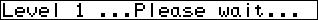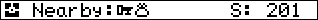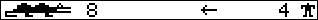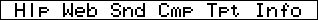##### Sharp PC-1500 : DungeonQuest game

"DungeonQuest" for Sharp PC-1500 +8 KB.
Author: Tralornik.
BASIC program - 7537 bytes.

In this pocket-size RPG, you explore a dungeon for gems and keys, while fighting horrible beasts. At each level, you need to find the 7 keys and then get to the exit... The game justs fits in an 8 KB RAM module.

Note: The program includes a "LOCK" statement to prevent you from accidently messing up the code. If you want to switch the PC-1500 to PRO mode, you need to type UNLOCK <ENTER> after stopping the program.

Start:
After you type RUN, the program displays a little banner, asks wheter you want sound (type Y or N), and then builds the dungeon. This will take approx 1mn15 (see screen 1).Break/Resume:
After the dungeon is constructed, you can BREAK and turn off the game, and resume it by typing GOTO "RESUME" <ENTER>. (Do not press break when the program is creating the dungeon.)
Display:
On the left, the PC1500 displays a top-down view of the room where you are, with the possible exits. A dot appears at the center of the room if you have already visited it. "Nearby:" indicates the objects/monsters in the adjacent rooms, if any. On the right is your strengh (S) (see screen 2).Displacements:
...8...
.4 + 6.
...2...

Each move costs 1 point in strength (up to level 10) , then 2 points (from level 11 to 20), and so on.
As you find silver, gold, gems, etc, they are picked up automatically (or fallen into in the case of traps).
Fight (5 key):
When you encounter a beast, you are shown on the right and the beast on the left (see screen 3). Each of you has a number randomly changing...Press the <5> key to launch an arrow and your number must match or beat the beast's number. (Your number is from 1 to 8, the beast is from 4 to 8). You win treasure and strength if you kill the beast quickly. You loose strengh if you take to many tries.Pressing F1 shows what other F-keys do (see screen 4)Web (F2 key) - Author web site.
Sound (F3 key) - Turn sound on/off.
Compass (F4 key) - Indicate the direction of the exit.
Transport (F5 key)
A Transport allows you to set a room, then move anywhere and then "transport" back to the room in one move. Quite useful when you find the exit but don't have all the keys to exit.
Info (F6 key) - Display treasure points, keys found, and current level.

BASIC program.

1 "DUNGEONQUEST"CLEAR :DIM A\$(30,30)*1:RANDOM :DIM K(20),L(20)
2 WAIT 200:PRINT "Dungeon Quest Ver1.3 by"
3 PRINT "BattleQuest.com, Inc."
4 PRINT "(c)Copyright 1988-2002":PRINT "BattleQuest.com, Inc."
5 SC=0:ST=200:Q=1:Z\$="":X1\$="NULL":WAIT 75:LOCK
10 REM MAIN PROGRAM
15 GOSUB "SOUND"
20 G=0:GOSUB "SETUP"
30 "RESUME"WAIT 0:GOSUB "DIRECTION"
35 GOSUB "SCREEN":IF A\$(X,Y)<>"B"AND A\$(X,Y)<>"E"AND A\$(X,Y)<>"e"THEN LET A\$(X,Y)="O"
40 GOSUB "MOVEMENT"
45 ST=ST-(1*(INT (Q/10)+1))
50 IF A\$(X,Y)="K"THEN GOSUB "KEY"
60 IF A\$(X,Y)="G"THEN GOSUB "GOLD"
70 IF A\$(X,Y)="S"THEN GOSUB "SILVER"
80 IF A\$(X,Y)="J"THEN GOSUB "JEWELS"
85 IF A\$(X,Y)="B"THEN GOSUB "BEAST"
90 IF A\$(X,Y)="E"OR A\$(X,Y)="e"THEN GOSUB "EXIT"
95 IF G=7AND (A\$(X,Y)="E"OR A\$(X,Y)="e")THEN GOTO 20
100 IF A\$(X,Y)="T"THEN GOSUB "TRAP"
110 IF ST<=0THEN GOTO "GAMEOVER"
120 GOTO 30
900 REM END OF MAIN PROGRAM
901 REM
902 REM
903 REM
1000 "SETUP"PRINT "Constructing Dungeon ":PRINT "Level";Q;" ...Please wait..."
1002 X1\$="NULL"
1005 FOR X=1TO 30:FOR Y=1TO 30:A\$(X,Y)="X":NEXT Y:NEXT X
1007 Z1=Q:IF Z1>10THEN LET Z1=10
1010 X=8+Z1:Y=X
1020 A\$(X,Y)=" "
1022 K(1)=Z1+8:L(1)=Z1+8
1024 FOR I=2TO 8+Z1
1026 K(I)=RND (16+Z1)+2
1028 L(I)=RND (16+Z1)+2
1030 N\$="TRUE"
1032 FOR M=1TO I
1034 IF K(I)=(K(M)+1)OR K(I)=(K(M)-1)THEN LET N\$="FALSE"
1036 IF L(I)=(L(M)+1)OR L(I)=(L(M)-1)THEN LET N\$="FALSE"
1038 NEXT M
1040 IF N\$="FALSE"THEN GOTO 1026
1042 NEXT I
1044 X=K(1):Y=L(1)
1046 FOR Z=1TO 7+Z1
1048 P=SGN (K(Z+1)-K(Z))
1050 IF P=0THEN LET P=1
1052 FOR X=K(Z)TO K(Z+1)STEP P
1054 GOSUB "ROOM"
1056 NEXT X
1060 X=K(Z+1)
1062 P=SGN (L(Z+1)-L(Z))
1064 IF P=0THEN LET P=1
1066 FOR Y=L(Z)TO L(Z+1)STEP P
1068 GOSUB "ROOM"
1070 NEXT Y
1072 Y=L(Z+1)
1074 NEXT Z
1076 X=K(8+Z1):Y=L(8+Z1)
1078 IF X=8+Z1AND Y=8+Z1THEN GOTO "SETUP"
1080 A\$(X,Y)="E":GOSUB "PLACEKEYS":X=8+Z1:Y=X:A\$(X,Y)=" "
1085 BEEP 5:PAUSE "Construction Complete...":BEEP 5
1090 RETURN
2090 REM END OF SETUP
2091 REM
2092 REM
2093 REM
2100 "ROOM"
2110 M=RND (100)
2120 IF M<3THEN LET A\$(X,Y)="J":RETURN
2130 IF M>2AND M<6THEN LET A\$(X,Y)="G":RETURN
2140 IF M>5AND M<10THEN LET A\$(X,Y)="S":RETURN
2150 IF M>9AND M<12THEN LET A\$(X,Y)="T":RETURN
2160 IF M>11AND M<(27+Z1)THEN LET A\$(X,Y)="B":RETURN
2170 A\$(X,Y)=" "
2180 RETURN
2190 REM END OF ROOM
2191 REM
2192 REM
2193 REM
2200 "PLACEKEYS"
2210 FOR I=1TO 7
2220 M=RND (11+Z1)+2:N=RND (11+Z1)+2
2230 IF (M=8+Z1AND N=8+Z1)OR A\$(M,N)="X"OR A\$(M,N)="K"OR A\$(M,N)="E"THEN GOTO 2220
2240 A\$(M,N)="K"
2250 NEXT I
2260 RETURN
2290 REM END OF PLACEKEYS
2291 REM
2292 REM
2293 REM
3000 "DIRECTION"B1\$="":B2\$=""
3010 A1\$="7F7F":A2\$="63":A3\$="6":A4\$="3":A5\$="7F7F00":A9\$=A\$(X,Y)
3015 IF A9\$="O"OR A9\$="e"THEN LET A4\$="B"
3020 IF A\$(X-1,Y)<>"X"THEN LET A1\$="7777"
3030 IF A\$(X+1,Y)<>"X"THEN LET A5\$="777700"
3040 IF A\$(X,Y+1)<>"X"THEN LET A3\$="0"
3050 IF A\$(X,Y-1)<>"X"THEN LET A4\$="0"
3055 IF A\$(X,Y-1)<>"X"AND (A9\$="O"OR A9\$="e")THEN LET A4\$="8"
3060 B1\$=A1\$+A2\$+A3\$+A4\$+A2\$+A5\$
3070 RETURN
3900 REM END OF DIRECTION
3901 REM
3902 REM
3903 REM
4000 "GOLD"WAIT 0:CURSOR 15:PRINT SC;:GCURSOR 82:GPRINT "06427E0F7E4206";
4005 FOR M=1TO 71:GCURSOR M:GPRINT "00304A4546454A3000":NEXT M:CURSOR 15:PRINT SC+100*Q
4007 CURSOR 1:PRINT "GOLD"
4010 FOR I=66TO 33STEP -1:BEEP 1,I,20:NEXT I
4020 SC=SC+(100*Q)
4030 RETURN
4900 REM END OF GOLD
4901 REM
4902 REM
4903 REM
5000 "SILVER"WAIT 0:CURSOR 15:PRINT SC;:GCURSOR 82:GPRINT "06427E0F7E4206";
5005 FOR M=1TO 71:GCURSOR M:GPRINT "00242A7F2A7F2A1200":NEXT M:CURSOR 15:PRINT SC+50*Q
5007 CURSOR 1:PRINT "SILVER"
5010 FOR I=99TO 66STEP -1:BEEP 1,I,20:NEXT I
5020 SC=SC+(50*Q)
5030 RETURN
5900 REM END OF SILVER
5901 REM
5902 REM
5903 REM
6000 "JEWELS"WAIT 0:CURSOR 15:PRINT SC;:GCURSOR 82:GPRINT "06427E0F7E4206";
6005 FOR M=1TO 71:GCURSOR M:GPRINT "00040E152E7D2E150E0400":NEXT M:CURSOR 15:PRINT SC+150*Q
6007 CURSOR 1:PRINT "GEM"
6010 FOR I=33TO 1STEP -1:BEEP 1,I,20:NEXT I
6020 SC=SC+(150*Q)
6030 RETURN
6900 REM END OF JEWELS
6901 REM
6902 REM
6903 REM
7000 "TRAP"WAIT 0:CURSOR 15:PRINT SC;:GCURSOR 84:GPRINT "06427E0F7E4206";
7005 FOR M=1TO 144STEP 2:GCURSOR M:GPRINT "00000001017F404442417F010100";:NEXT M
7007 CURSOR 1:PRINT "TRAP"
7010 FOR I=1TO 99:BEEP 1,I,10:NEXT I
7020 ST=ST-INT (ST/5)
7025 A\$(X,Y)="O"
7030 X=8+Z1:Y=X
7040 RETURN
7900 REM END OF TRAP
7901 REM
7902 REM
7903 REM
8000 "BEAST"WAIT 0:PRINT ">>>>>>>>>> BEAST <<<<<<<<<":DI=0
8010 FOR II=1TO 2:FOR I=1TO 30:BEEP 1,I,10:NEXT I:NEXT II
8015 CLS
8020 GCURSOR 1:GPRINT "7050505058581E1E1F1F7F7E5E5C1C1E1E1F1F1F7E7E5C5C1C1C16151614141414";
8025 GCURSOR 149:GPRINT "06427E0F7E4206";
8029 IF Q>5GOTO 8033
8030 CURSOR 6:BE=RND (5)+3:YO=RND (8):PRINT BE;:CURSOR 22:PRINT YO;
8031 GOTO 8035
8033 CURSOR 6:BE=RND (4)+4:YO=RND (8):PRINT BE;:CURSOR 22:PRINT YO;
8035 IF INKEY\$ ="5"THEN 8050
8040 GOTO 8030
8050 FOR I=120TO 46STEP -3:GCURSOR I:GPRINT "081C2A0808080808000000"
8055 IF Q>5GOTO 8062
8060 BE=RND (5)+3:CURSOR 6:PRINT BE;:NEXT I
8061 GOTO 8064
8062 BE=RND (4)+4:CURSOR 6:PRINT BE;:NEXT I
8064 FOR I=1TO 50:NEXT I
8065 IF BE>YOTHEN LET DI=DI+3:GOTO 8015
8070 IF YO>=BETHEN FOR I=46TO 1STEP -3:GCURSOR I:GPRINT "081C2A0808080808000000":NEXT I:A\$(X,Y)="O"
8080 IF DI<9THEN CLS :PAUSE "You won";(9-DI)*10*Q;" in treasure":SC=SC+((9-DI)*10*Q)
8090 IF DI<9THEN CLS :PAUSE "You won";9-DI;" in strength":ST=ST+(9-DI):A\$(X,Y)=" "
8095 IF DI=9THEN CLS :PAUSE "You did not gain nor lose."
8100 IF DI>9THEN CLS :PAUSE "You lost";DI-9;" in strength":ST=ST-(DI-9)
8150 A\$(X,Y)=" "
8200 RETURN
8900 REM END OF BEAST
8901 REM
8902 REM
8903 REM
9000 "GAMEOVER"PRINT "You have died !!!":FOR I=1TO 100:BEEP 1,I,20:NEXT I
9010 WAIT 200:PRINT "Treasure: ";SC
9020 INPUT "Play again? (Y/N/T) ";Z\$
9030 IF Z\$<>"Y"AND Z\$<>"N"AND Z\$<>"T"THEN PAUSE "Y-YES N-NO T-TREASURE":GOTO 9020
9040 IF Z\$="T"THEN GOTO 9010
9050 IF Z\$="Y"THEN GOTO 1
9060 WAIT :PRINT "Treasure: ";SC:END
9900 REM END OF GAMEOVER
9901 REM
9902 REM
9903 REM
10000 "SCREEN"CLS :WAIT 0
10010 N1\$=A\$(X-1,Y):N2\$=A\$(X+1,Y):N3\$=A\$(X,Y-1):N4\$=A\$(X,Y+1)
10020 REM
10025 GCURSOR 1:GPRINT B1\$;:PRINT " Nearby:";
10030 IF N1\$="K"OR N2\$="K"OR N3\$="K"OR N4\$="K"THEN GPRINT "3E223E0C2C3C2C0400";
10040 IF N1\$="G"OR N2\$="G"OR N3\$="G"OR N4\$="G"THEN GPRINT "304A4546454A30000";
10050 IF N1\$="S"OR N2\$="S"OR N3\$="S"OR N4\$="S"THEN GPRINT "242A7F2A7F2A1200";
10060 IF N1\$="J"OR N2\$="J"OR N3\$="J"OR N4\$="J"THEN GPRINT "040E152E7D2E150E0400";
10070 IF N1\$="E"OR N2\$="E"OR N3\$="E"OR N4\$="E"THEN GPRINT "407F41414149417F4000";
10080 IF N1\$="e"OR N2\$="e"OR N3\$="e"OR N4\$="e"THEN GPRINT "407F41414149417F4000";
10090 IF N1\$="T"OR N2\$="T"OR N3\$="T"OR N4\$="T"THEN GPRINT "01017F404442417F010100";
10100 IF N1\$="B"OR N2\$="B"OR N3\$="B"OR N4\$="B"THEN GPRINT "3E3C1615161414141400";
10110 CURSOR 19:PRINT "S:";ST
10115 WAIT 75
10120 RETURN
10900 REM END OF SCREEN
10901 REM
10902 REM
10903 REM
11000 "MOVEMENT"
11010 Z\$=INKEY\$ :IF Z\$<>"8"AND Z\$<>"6"AND Z\$<>"2"AND Z\$<>"4"AND (ASC Z\$<16OR ASC Z\$>23)THEN GOTO 11010
11013 IF Z\$=CHR\$ 17THEN WAIT 200:PRINT " Hlp Web Snd Cmp Tpt Info":WAIT 75:GOSUB "SCREEN":GOTO 11000
11014 IF Z\$=CHR\$ 18THEN WAIT 200:PRINT "Web: www.BattleQuest.com":WAIT 75:GOSUB "SCREEN":GOTO 11000
11015 IF Z\$=CHR\$ 19THEN GOSUB "SOUND":GOSUB "SCREEN":GOTO 11000
11020 IF Z\$="4"AND A\$(X-1,Y)<>"X"THEN LET X=X-1:NM\$="WEST":GOTO 11100
11025 IF Z\$=CHR\$ 22THEN WAIT 200:PRINT "TR:";SC;" K:";G;" Lvl:";Q:GOSUB "SCREEN":WAIT 75:GOTO 11000
11030 IF Z\$="6"AND A\$(X+1,Y)<>"X"THEN LET X=X+1:NM\$="EAST":GOTO 11100
11035 IF Z\$=CHR\$ 21THEN GOSUB "TRANSPORT":GOTO 11070
11040 IF Z\$="8"AND A\$(X,Y-1)<>"X"THEN LET Y=Y-1:NM\$="NORTH":GOTO 11100
11045 IF Z\$=CHR\$ 20THEN GOSUB "COMPASS":GOSUB "SCREEN":GOTO 11000
11050 IF Z\$="2"AND A\$(X,Y+1)<>"X"THEN LET Y=Y+1:NM\$="SOUTH":GOTO 11100
11060 CLS :PAUSE "You walked into a wall..."
11070 CLS :RETURN
11100 GCURSOR 1
11110 IF NM\$="SOUTH"THEN GPRINT "0018207F201800";
11120 IF NM\$="NORTH"THEN GPRINT "000C027F020C00";
11140 IF NM\$="WEST"THEN GPRINT "081C2A2A080808";
11150 IF NM\$="EAST"THEN GPRINT "0808082A2A1C08";
11160 GOTO 11070
11900 REM END OF MOVEMENT
12000 "KEY"PRINT "********** KEY ***********"
12010 BEEP 5:G=G+1
12020 PRINT "You found key No.";G;" of 7"
12030 PRINT "Bonus";G*10;" in treasure"
12040 PRINT "Bonus";G*2;" in strength"
12050 SC=SC+(G*10):ST=ST+(G*2)
12055 IF G=7THEN PRINT "Find the door to exit Lvl"
12060 RETURN
12900 REM END OF KEY
12901 REM
12902 REM
12903 REM
13000 "EXIT"PAUSE "You are at the exit":WAIT 75
13010 IF G<7THEN PAUSE "but you need";7-G;" more key(s).":A\$(X,Y)="e"
13020 IF G>=7THEN GOTO 13040
13030 RETURN
13040 PRINT "Bonus ";1000+(Q*500);" in treasure":BEEP 5
13050 PRINT "Bonus ";50+(Q*10);" in strength":BEEP 5
13060 SC=SC+1000+(Q*500):ST=ST+50+(Q*10)
13065 Q=Q+1
13070 RETURN
13900 REM END OF EXIT
13901 REM
13902 REM
13903 REM
14000 "TRANSPORT"WAIT 0
14010 PRINT "Transport, Set or Exit? ":M\$=INKEY\$ :IF M\$=""THEN 14010
14015 IF M\$<>"T"AND M\$<>"S"AND M\$<>"E"THEN 14010
14016 IF M\$="E"THEN RETURN
14020 IF M\$="T"AND X1\$<>"NULL"THEN PAUSE "Transporting...":X=X1:Y=Y1
14030 IF M\$="T"AND X1\$="NULL"THEN PAUSE "Transport has not been set"
14040 IF M\$="S"THEN PAUSE "Transport has been set.":X1=X:Y1=Y:X1\$="HERE"
14050 RETURN
14900 REM END OF TRANSPORT
14901 REM
14902 REM
14903 REM
15000 "COMPASS"WAIT 75:PRINT "Compass? (Y or N)":Z\$=INKEY\$ :IF Z\$<>"Y"AND Z\$<>"N"THEN GOTO 15000
15010 IF Z\$="N"THEN RETURN
15015 IF ST<((Z1*5)+1)THEN PAUSE "You need more strength.":RETURN
15020 PRINT "The exit is ";
15030 IF Y>L(Z1+8)THEN PRINT "North";
15040 IF Y<L(Z1+8)THEN PRINT "South";
15050 IF X<K(Z1+8)THEN PRINT "East";
15060 IF X>K(Z1+8)THEN PRINT "West";
15070 PRINT "."
15075 ST=ST-(Z1*5)
15080 RETURN
15990 REM END OF COMPASS
15991 REM
15992 REM
15999 REM
16000 REM
16010 REM
16015 REM SOUND
16020 "SOUND"
16025 PRINT "Sound? (Y or N)"
16030 Z\$=INKEY\$ :IF Z\$<>"Y"AND Z\$<>"N"THEN GOTO 16030
16035 IF Z\$="Y"THEN BEEP ON
16040 IF Z\$="N"THEN BEEP OFF
16050 RETURN
59990 REM
59999 REM PRINT MAP
60000 FOR X=1TO 30:FOR Y=1TO 30:LPRINT A\$(X,Y);:NEXT Y:LPRINT :NEXT X
60001 END